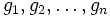Multiplication table of a finite group

VIEW: Definitions built on this | Facts about this: (facts closely related to Multiplication table of a finite group, all facts related to Multiplication table of a finite group) |Survey articles about this | Survey articles about definitions built on this
VIEW RELATED: Analogues of this | Variations of this | Opposites of this |[SHOW MORE]

Definition

Definition with symbols

Let$G$ be a finite group of order$n$. Then, the multiplication table of$G$ is a$n \times n$ matrix described as follows:

• Label the elements of$G$ as$g_1,g_2,\ldots,g_n$ such that$g_1$ is the identity element.
• Now, in the$(i,j)^{th}$ entry of the matrix, put the element$g_ig_j$

Note that under this definition, the multiplication table is an$n \times n$ matrix with entries drawn from$G$. We can, however, change this to a$n \times n$ matrix with entries drawn from$1,2,\ldots,n$ by simply replacing$g \in G$ by the$k$ for which$g = g_k$.

The latter interpretation makes the multiplication table into a purely combinatorial object. We shall use this latter interpretation.

Clearly, the multiplication table determines the group uniquely.

We can also construct the multiplication table of any finite magma in the same way.

Important note on commutativity

In a non-Abelian group, interchanging the role of rows and columns changes the multiplication table. Thus, we need to follow a convention (the convention here is that the row element is multiplied on the left and the column element is on the right).

For an Abelian group, the group operation is more typically termed addition, and the multiplication table is thus termed an addition table.

Examples

The trivial group

Here's how the multiplication table of the trivial group looks:

Element$e$ (identity element)$e$$e$

A group of order two

Element$e$ (identity element)$x$ (non-identity element)$e$$e$$x$$x$$x$$e$

A non-Abelian group of order six

Below is the multiplication table of a non-Abelian group of order six. This is actually the group of permutations on three letters (following the cycle decomposition notation).

Properties

Latin square

The multiplication table of a finite group is a Latin square, when we label the entries$1,2,\ldots,n$. In other words, every row contains each element exactly once and every column contains each element exactly once.

In fact, the multiplication table of a finite magma is a latin square if and only if that magma is a quasigroup. Groups are indeed quasigroups.

Identity element

If we choose the label$1$ for the identity element, the first row and first column are both$1,2,\dots,n$.

Commutativity yields symmetric Latin square

The multiplication table of a finite group (and more generally, of any finite magma) is a symmetric matrix if and only if the binary operation is commutative. In the case of groups, this boils down to saying that the group is Abelian.

How inverses affect the Latin square

The existence of two-sided inverses means that, even if the Latin square is not symmetric, it is symmetric with respect to the identity element. In other words, if the$(i,j)^{th}$ entry is the identity element, then so is the$(j,i)^{th}$ entry.

How associativity affects the Latin square

Not every Latin square arises from a group, even if the first row and first column are the identity element, and even if every element has a two-sided inverse. The problem could be the lack of associativity. The constraint that associativity puts on a Latin square is not easy to state in visual terms.

Automorphisms of the group

Let$P$ be the permutation matrix corresponding to an automorphism of$G$. If$M$ denotes the multiplication table matrix for$G$, we have:$PMP^t = M$

This is equivalent to saying that$M$ commutes with$P$.

In other words, the centralizer of$M$ in$GL_n(\mathbb{Z})$ contains all permutation matrices arising from automorphisms of$G$.

Computation

GAP command

The GAP command for computing the multiplication table of a finite group is: PrintTable

The PrintTable command computes the multiplication table of a finite group. This command requires the package Sonata, that can be loaded with the command LoadPackage("sonata");.